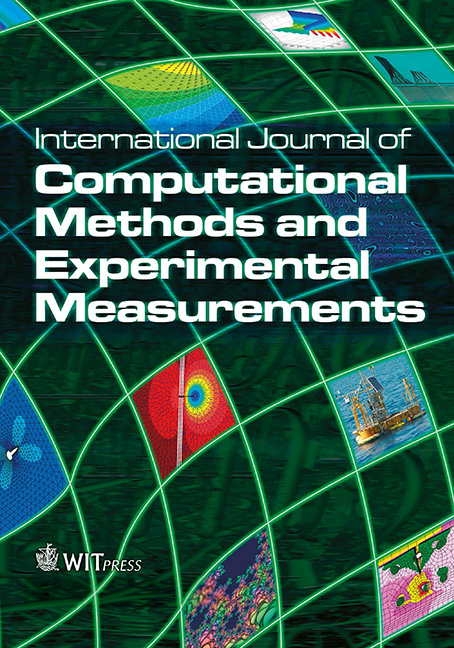WIT Press

FINER THEORETICAL ASPECTS OF THE DRIFT FLUX FAMILY OF EQUATIONS FOR SIMULATING AVERAGED VOLUME FRACTION IN MULTIPHASE FLOWS

Price

Free (open access)Volume

Volume 6 (2018), Issue 2

Pages

10

Page Range

240 - 250

Paper DOI

10.2495/CMEM-V6-N2-240-250

WIT Press

Author(s)

A. S. NAGOO & M. M. SHARMA

Abstract

The drift flux formulation is widely regarded as being amenable to computationally efficient calculation of averaged slip between phases (i.e. averaged volume fraction) in multiphase flows. However, contrary to the common perception in practice that this formulation provides two simple data-fitting parameters for describing averaged slip, it is shown that these parameters cannot be mathematically disassociated from each other and are intricately related to both the averaged and local phase velocities and volume fractions. This derived fact also provides a rational explanation for the extreme difficulty in the past to resolve the influence of the local lateral distribution (multidimensional) effects of the dispersed phase in the flow on the averaged flow characteristics. Of further significance from this derivation is the fact that correlations for drift flux parameters in which these terms are expressed explicitly from each other cannot claim a better understanding of the flow physics unless they capture this inherent analytical bi-directional dependency. All of the multiphase flow variables that affect the drift velocity parameter also affect the distribution parameter. In terms of simulation applications, the new analytical derivations presented enable a re-formulation and unification of prior volume fraction models and an explanation for field observations of why a reduction in the drift velocity parameter will lead to reduction in the distribution parameter. As much as numerical computing and simulation methods are invaluable tools, the results of this work show that it is equally important to explore their mathematical basis and scientific understanding that can help to advance more efficient simulation of multiphase flow behaviors in the field.

Keywords

analytical prediction, drift flux parameters, fractional flow theory, multiphase flow simulation, pipe, pipeline, slip ratio, volume fraction, wellbore